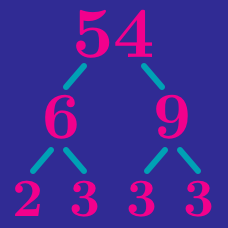Number Theory

# Prime Factor Trees

If you complete this factor tree, what number must replace the question mark?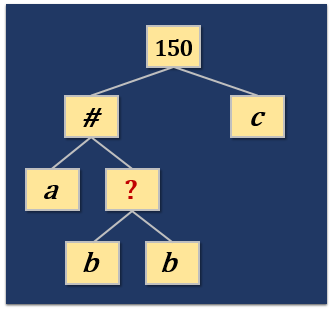Details and assumptions:

$a, b$ and $c$ are prime numbers.

How many of these trees are correct prime factor trees for 28?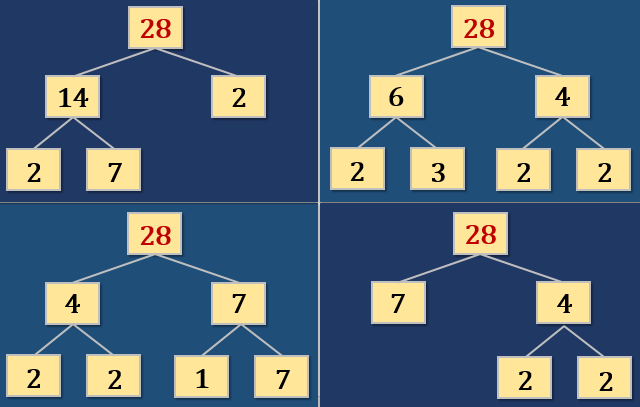If $x$ and $y$ are primes, what is the relationship between $A$ and $B?$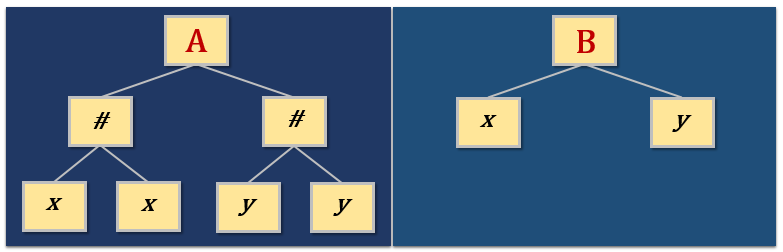Given their structures, could both of these trees be prime factor trees for the same number?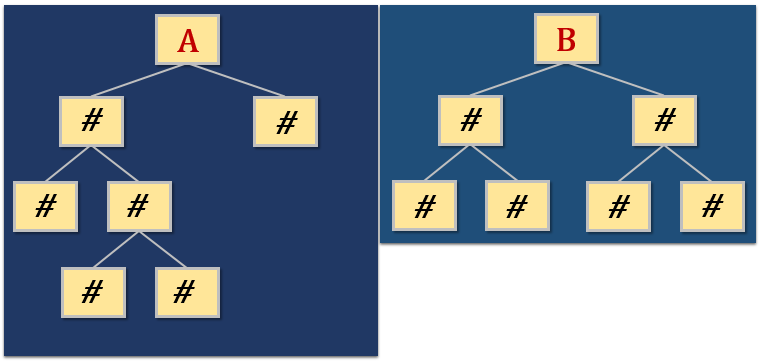Which of these numbers has a prime factor tree that's shaped like the one below?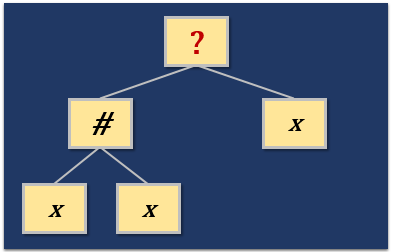Details and assumptions:

$x$ is a prime number.

×